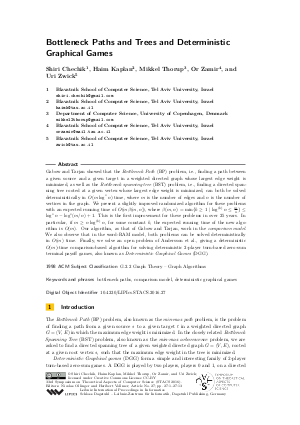Document# Bottleneck Paths and Trees and Deterministic Graphical Games

### Authors Shiri Chechik, Haim Kaplan, Mikkel Thorup, Or Zamir, Uri Zwick## File

LIPIcs.STACS.2016.27.pdf
• Filesize: 0.65 MB
• 13 pages

## Cite As

Shiri Chechik, Haim Kaplan, Mikkel Thorup, Or Zamir, and Uri Zwick. Bottleneck Paths and Trees and Deterministic Graphical Games. In 33rd Symposium on Theoretical Aspects of Computer Science (STACS 2016). Leibniz International Proceedings in Informatics (LIPIcs), Volume 47, pp. 27:1-27:13, Schloss Dagstuhl - Leibniz-Zentrum für Informatik (2016)
https://doi.org/10.4230/LIPIcs.STACS.2016.27

## Abstract

Gabow and Tarjan showed that the Bottleneck Path (BP) problem, i.e., finding a path between a given source and a given target in a weighted directed graph whose largest edge weight is minimized, as well as the Bottleneck spanning tree (BST) problem, i.e., finding a directed spanning tree rooted at a given vertex whose largest edge weight is minimized, can both be solved deterministically in O(m * log^*(n)) time, where m is the number of edges and n is the number of vertices in the graph. We present a slightly improved randomized algorithm for these problems with an expected running time of O(m * beta(m,n)), where beta(m,n) = min{k >= 1 | log^{(k)}n <= m/n } <= log^*(n) - log^*(m/n)+1. This is the first improvement for these problems in over 25 years. In particular, if m >= n * log^{(k)} * n, for some constant k, the expected running time of the new algorithm is O(m). Our algorithm, as that of Gabow and Tarjan, work in the comparison model. We also observe that in the word-RAM model, both problems can be solved deterministically in O(m) time. Finally, we solve an open problem of Andersson et al., giving a deterministic O(m)-time comparison-based algorithm for solving deterministic 2-player turn-based zero-sum terminal payoff games, also known as Deterministic Graphical Games (DGG).
##### Keywords
• bottleneck paths
• comparison model
• deterministic graphical games

## Metrics

• Access Statistics
• Total Accesses (updated on a weekly basis)
0

## References

1. D. Andersson, K.A. Hansen, P.B. Miltersen, and T.B. Sørensen. Deterministic graphical games revisited. Journal of Logic and Computation, 22(2):165-178, 2010.2. H. Björklund, S. Sandberg, and S. Vorobyov. Memoryless determinacy of parity and mean payoff games: a simple proof. Theoretical Computer Science, 310(1-3):365-378, 2004.3. H. Björklund and S. Vorobyov. Combinatorial structure and randomized subexponential algorithms for infinite games. Theoretical Computer Science, 349(3):347-360, 2005.4. M. Blum, R.W. Floyd, V. Pratt, R.L. Rivest, and R.E. Tarjan. Time bounds for selection. Journal of Computer and System Sciences, 7(4):448-461, 1973.5. P.M. Camerini. The min-max spanning tree problem and some extensions. Information Processing Letters, 7(1):10-14, 1978. URL: http://dx.doi.org/10.1016/0020-0190(78)90030-3.
6. B. Chazelle. A minimum spanning tree algorithm with inverse-Ackermann type complexity. Journal of the ACM, 47(6):1028-1047, 2000.7. E.W. Dijkstra. A note on two problems in connexion with graphs. Numerische Mathematik, 1:269-271, 1959.8. R. Duan and S. Pettie. Fast algorithms for (max, min)-matrix multiplication and bottleneck shortest paths. In Proc. of 20th SODA, pages 384-391, 2009.9. J. Edmonds and D.R. Fulkerson. Bottleneck extrema. Journal of Combinatorial Theory, 8(3):299-306, 1970.10. J. Edmonds and R.M. Karp. Theoretical improvements in algorithmic efficiency for network flow problems. Journal of the ACM, 19(2):248-264, 1972.11. A. Ehrenfeucht and J. Mycielski. Positional strategies for mean payoff games. International Journal of Game Theory, 8:109-113, 1979.12. E.A. Emerson and C. Jutla. Tree automata, μ-calculus and determinacy. In Proc. of 32nd FOCS, pages 368-377, 1991.13. M.L. Fredman and R.E. Tarjan. Fibonacci heaps and their uses in improved network optimization algorithms. Journal of the ACM, 34(3):596-615, 1987.14. M.L. Fredman and D.E. Willard. Surpassing the information-theoretic bound with fusion trees. Journal of Computer and System Sciences, 47(3):424-436, 1993.15. H.N. Gabow, Z. Galil, T.H. Spencer, and R.E. Tarjan. Efficient algorithms for finding minimum spanning trees in undirected and directed graphs. Combinatorica, 6:109-122, 1986.16. H.N. Gabow and R.E. Tarjan. Algorithms for two bottleneck optimization problems. Journal of Algorithms, 9(3):411-417, 1988.17. V.A. Gurvich, A.V. Karzanov, and L.G. Khachiyan. Cyclic games and an algorithm to find minimax cycle means in directed graphs. USSR Computational Mathematics and Mathematical Physics, 28:85-91, 1988.18. Y. Han and M. Thorup. Integer sorting in O(n√log log n) expected time and linear space. In Proc. of 43rd FOCS, pages 135-144, 2002.19. T.C. Hu. The maximum capacity route problem. Operations Research, 9(6):898-900, 1961.20. M. Jurdziński, M. Paterson, and U. Zwick. A deterministic subexponential algorithm for solving parity games. SIAM Journal on Computing, 38(4):1519-1532, 2008.21. D.R. Karger, P.N. Klein, and R.E. Tarjan. A randomized linear-time algorithm to find minimum spanning trees. Journal of the ACM, 42:321-328, 1995.22. J. Matoušek. Computing dominances in Eⁿ. Information Processing Letters, 38(5):277-278, 1991. URL: http://dx.doi.org/10.1016/0020-0190(91)90071-O.
23. A.P. Punnen. A fast algorithm for a class of bottleneck problems. Computing, 56(4):397-401, 1996. URL: http://dx.doi.org/10.1007/BF02253463.
24. A. Shapira, R. Yuster, and U. Zwick. All-pairs bottleneck paths in vertex weighted graphs. Algorithmica, 59:621-633, 2011.25. V. Vassilevska, R. Williams, and R. Yuster. All pairs bottleneck paths and max-min matrix products in truly subcubic time. Theory of Computing, 5(1):173-189, 2009.26. A. Washburn. Deterministic graphical games. Journal of Mathematical Analysis and Applications, 153(1):84-96, 1990.27. E. Zermelo. Über eine anwendung der mengenlehre auf die theorie des schachspiels. In Proceedings of the Fifth International Congress of Mathematicians, pages 501-–504, 1913.28. U. Zwick and M.S. Paterson. The complexity of mean payoff games on graphs. Theoretical Computer Science, 158(1-2):343-359, 1996.X

Feedback for Dagstuhl Publishing